5-demicube

﻿
5-demicube
Demipenteract
(5-demicube)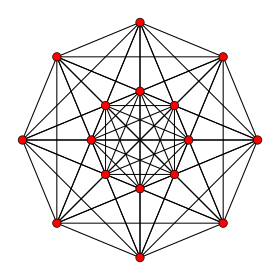Petrie polygon projection
Type Uniform 5-polytope
Family (Dn) 5-demicube
Families (En) k21 polytope
1k2 polytope
Coxeter symbol 121
Schläfli symbol {31,2,1}
h{4,3,3,3}
s{2,2,2,2}
Coxeter-Dynkin diagram4-faces 26 10 {31,1,1}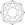16 {3,3,3}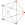Cells 120 40 {31,0,1}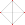80 {3,3}Faces 160 {3}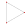Edges 80
Vertices 16
Vertex figure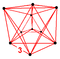rectified 5-cell
Petrie polygon Octagon
Symmetry group D5, [34,1,1] = [1+,4,33]
+
Properties convex

In five dimensional geometry, a demipenteract or 5-demicube is a semiregular 5-polytope, constructed from a 5-hypercube (penteract) with alternated vertices deleted.

It was discovered by Thorold Gosset. Since it was the only semiregular 5-polytope (made of more than one type of regular hypercell), he called it a 5-ic semi-regular.

Coxeter named this polytope as 121 from its Coxeter-Dynkin diagram, which has branches of length 2, 1 and 1 with a ringed node on one of the short branches. It exists in the k21 polytope family as 121 with the Gosset polytopes: 221, 321, and 421.

Cartesian coordinates

Cartesian coordinates for the vertices of a demipenteract centered at the origin and edge length 2√2 are alternate halves of the penteract:

(±1,±1,±1,±1,±1)

with an odd number of plus signs.

Projected images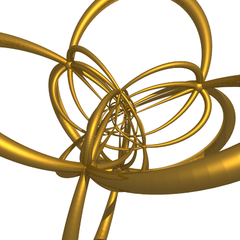Perspective projection.

Images

orthographic projections
Coxeter plane B5
Graph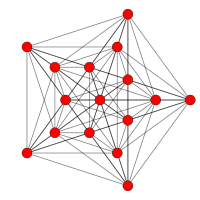Dihedral symmetry [10/2]
Coxeter plane D5 D4
Graph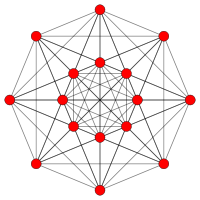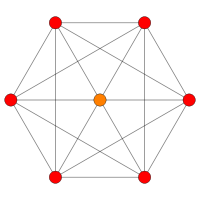Dihedral symmetry  
Coxeter plane D3 A3
Graph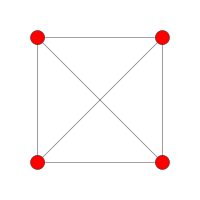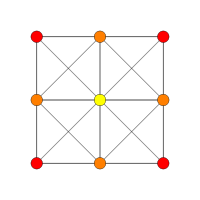Dihedral symmetry  

Related polytopes

It is a part of a dimensional family of uniform polytopes called demihypercubes for being alternation of the hypercube family.

There are 23 uniform polytera (uniform 5-polytope) that can be constructed from the D5 symmetry of the demipenteract, 8 of which are unique to this family, and 15 are shared within the penteractic family.

Wikimedia Foundation. 2010.

Look at other dictionaries:

• 6-demicube — Demihexeract (6 demicube) Petrie polygon projection Type Uniform 6 polytope Family demihypercube Schläfli symbol {3,33,1} h{4,3,3,3,3} s{2,2,2,2,2} …   Wikipedia

• 7-demicube — Demihepteract (7 demicube) Petrie polygon projection Type Uniform 7 polytope Family demihypercube Coxeter symbol 141 Schläfli symbol …   Wikipedia

• 9-demicube — Demienneract (9 demicube) Petrie polygon Type Uniform 9 polytope Family demihypercube Coxeter symbol 161 Schläfli symbol …   Wikipedia

• 8-demicube — Demiocteract (8 demicube) Petrie polygon projection Type Uniform 8 polytope Family demihypercube Coxeter symbol 151 …   Wikipedia

• 10-demicube — Demidekeract (10 demicube) Petrie polygon projection Type Uniform 10 polytope Family demihypercube Coxeter symbol 171 Schläfli symbol …   Wikipedia

• Demihypercube — Not to be confused with Hemicube (geometry). Alternation of the n cube yields one of two n demicubes, as in this 3 dimensional illustration of the two tetrahedra that arise as the 3 demicubes of the 3 cube. In geometry, demihypercubes (also… …   Wikipedia

• Coxeter group — In mathematics, a Coxeter group, named after H.S.M. Coxeter, is an abstract group that admits a formal description in terms of mirror symmetries. Indeed, the finite Coxeter groups are precisely the finite Euclidean reflection groups; the symmetry …   Wikipedia

• 16-cell — Regular hexadecachoron (16 cell) (4 orthoplex) Schlegel diagram (vertices and edges) Type Convex regular 4 polytope Schläfli symbo …   Wikipedia

• Cube — This article is about the geometric shape. For other uses, see Cube (disambiguation). Regular Hexahedron (Click here for rotating model) Type Platonic solid Elements F = 6, E = 12 V = 8 (χ = 2) …   Wikipedia

• Tetrahedron — For the academic journal, see Tetrahedron (journal). Regular Tetrahedron (Click here for rotating model) Type Platonic solid Elements F = 4, E = 6 V = 4 (χ = 2) Faces by s …   Wikipedia